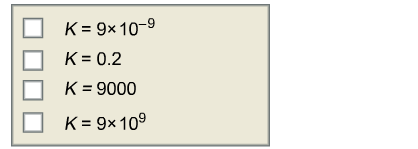Chemistry Practice Problems Chemical Equilibrium Practice Problems Solution: For the reversible reactionA(g) ⇌ B(g)which k valu...

🤓 Based on our data, we think this question is relevant for Professor Blue's class at UK.

# Solution: For the reversible reactionA(g) ⇌ B(g)which k values would indicate that there is more B than A at equilibrium?

###### Problem

For the reversible reaction

A(g) ⇌ B(g)

which k values would indicate that there is more B than A at equilibrium?View Complete Written SolutionChemical Equilibrium

Chemical Equilibrium

#### Q. Calculate the value of Kp for the equation. C(s) + CO2(g) ⇌ 2CO(g)                              Kp=? given that at a certain temperature C(s) + 2H2...

Solved • Tue Feb 13 2018 13:07:27 GMT-0500 (EST)

Chemical Equilibrium

#### Q. A + 2B → 2C      Kc = 2.392C → D               Kc = 0.176Calculate the value of the equilibrium constant for the reactionD → A + 2B         Kc = ?

Solved • Fri Dec 22 2017 11:00:31 GMT-0500 (EST)

Chemical Equilibrium

#### Q. Calculate the value of the equilibrium constant K for the hypothetical reaction2D → A + 2Bfrom the following information:A + 2B → C   Kc = 3.3C → 2D  ...

Solved • Tue Dec 19 2017 11:15:15 GMT-0500 (EST)

Chemical Equilibrium

#### Q. For A + 2B → 2C  Kc= 2.79                    For 2C → D  Kc= 0.106Calculate the value of the equilibrium constant for the reaction:D → A + 2BKc=?

Solved • Tue Dec 19 2017 11:09:20 GMT-0500 (EST)